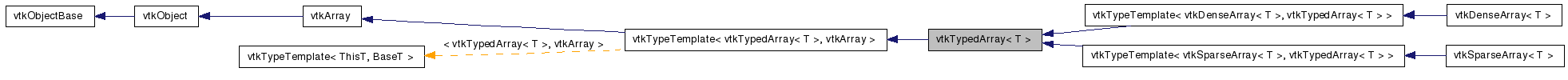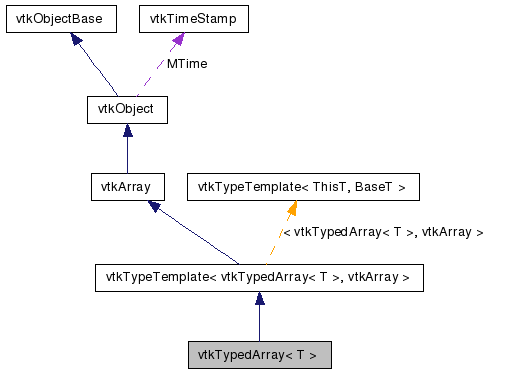# vtkTypedArray< T > Class Template Reference

`#include <vtkTypedArray.h>`

Inheritance diagram for vtkTypedArray< T >:[legend]
Collaboration diagram for vtkTypedArray< T >:[legend]

## Detailed Description

### template<typename T> class vtkTypedArray< T >

Provides a type-specific interface to N-way arrays.

vtkTypedArray provides an interface for retrieving and updating data in an arbitrary-dimension array. It derives from vtkArray and is templated on the type of value stored in the array.

Methods are provided for retrieving and updating array values based either on their array coordinates, or on a 1-dimensional integer index. The latter approach can be used to iterate over the values in an array in arbitrary order, which is useful when writing filters that operate efficiently on sparse arrays and arrays that can have any number of dimensions.

Special overloaded methods provide simple access for arrays with one, two, or three dimensions.

vtkArray, vtkDenseArray, vtkSparseArray
Thanks:
Tests:
vtkTypedArray (Tests)

Definition at line 58 of file vtkTypedArray.h.

## Public Member Functions

void PrintSelf (ostream &os, vtkIndent indent)
virtual vtkVariant GetVariantValue (const vtkArrayCoordinates &coordinates)
virtual vtkVariant GetVariantValueN (const vtkIdType n)
virtual void SetVariantValue (const vtkArrayCoordinates &coordinates, const vtkVariant &value)
virtual void SetVariantValueN (const vtkIdType n, const vtkVariant &value)
virtual void CopyValue (vtkArray *source, const vtkArrayCoordinates &source_coordinates, const vtkArrayCoordinates &target_coordinates)
virtual void CopyValue (vtkArray *source, const vtkIdType source_index, const vtkArrayCoordinates &target_coordinates)
virtual void CopyValue (vtkArray *source, const vtkArrayCoordinates &source_coordinates, const vtkIdType target_index)
virtual const T & GetValueN (const vtkIdType n)=0
virtual void SetValueN (const vtkIdType n, const T &value)=0
const T & GetValue (vtkIdType i)
const T & GetValue (vtkIdType i, vtkIdType j)
const T & GetValue (vtkIdType i, vtkIdType j, vtkIdType k)
virtual const T & GetValue (const vtkArrayCoordinates &coordinates)=0
void SetValue (vtkIdType i, const T &value)
void SetValue (vtkIdType i, vtkIdType j, const T &value)
void SetValue (vtkIdType i, vtkIdType j, vtkIdType k, const T &value)
virtual void SetValue (const vtkArrayCoordinates &coordinates, const T &value)=0

## Protected Member Functions

vtkTypedArray ()
~vtkTypedArray ()

## Constructor & Destructor Documentation

template<typename T>
 vtkTypedArray< T >::vtkTypedArray ( ) ` [inline, protected]`

Definition at line 110 of file vtkTypedArray.h.

template<typename T>
 vtkTypedArray< T >::~vtkTypedArray ( ) ` [inline, protected]`

Definition at line 111 of file vtkTypedArray.h.

## Member Function Documentation

template<typename T>
 void vtkTypedArray< T >::PrintSelf ( ostream & os, vtkIndent indent ) ` [virtual]`

Methods invoked by print to print information about the object including superclasses. Typically not called by the user (use Print() instead) but used in the hierarchical print process to combine the output of several classes.

Reimplemented from vtkArray.

Reimplemented in vtkDenseArray< T >, and vtkSparseArray< T >.

template<typename T>
 virtual vtkVariant vtkTypedArray< T >::GetVariantValue ( const vtkArrayCoordinates & coordinates ) ` [virtual]`

Returns the value stored in the array at the given coordinates. Note that the number of dimensions in the supplied coordinates must match the number of dimensions in the array.

Implements vtkArray.

template<typename T>
 virtual vtkVariant vtkTypedArray< T >::GetVariantValueN ( const vtkIdType n ) ` [virtual]`

Returns the n-th value stored in the array, where n is in the range [0, GetNonNullSize()). This is useful for efficiently visiting every value in the array. Note that the order in which values are visited is undefined, but is guaranteed to match the order used by vtkArray::GetCoordinatesN().

Implements vtkArray.

template<typename T>
 virtual void vtkTypedArray< T >::SetVariantValue ( const vtkArrayCoordinates & coordinates, const vtkVariant & value ) ` [virtual]`

Overwrites the value stored in the array at the given coordinates. Note that the number of dimensions in the supplied coordinates must match the number of dimensions in the array.

Implements vtkArray.

template<typename T>
 virtual void vtkTypedArray< T >::SetVariantValueN ( const vtkIdType n, const vtkVariant & value ) ` [virtual]`

Overwrites the n-th value stored in the array, where n is in the range [0, GetNonNullSize()). This is useful for efficiently visiting every value in the array. Note that the order in which values are visited is undefined, but is guaranteed to match the order used by vtkArray::GetCoordinatesN().

Implements vtkArray.

template<typename T>
 virtual void vtkTypedArray< T >::CopyValue ( vtkArray * source, const vtkArrayCoordinates & source_coordinates, const vtkArrayCoordinates & target_coordinates ) ` [virtual]`

Overwrites a value with a value retrieved from another array. Both arrays must store the same data types.

Implements vtkArray.

template<typename T>
 virtual void vtkTypedArray< T >::CopyValue ( vtkArray * source, const vtkIdType source_index, const vtkArrayCoordinates & target_coordinates ) ` [virtual]`

Overwrites a value with a value retrieved from another array. Both arrays must store the same data types.

Implements vtkArray.

template<typename T>
 virtual void vtkTypedArray< T >::CopyValue ( vtkArray * source, const vtkArrayCoordinates & source_coordinates, const vtkIdType target_index ) ` [virtual]`

Overwrites a value with a value retrieved from another array. Both arrays must store the same data types.

Implements vtkArray.

template<typename T>
 const T& vtkTypedArray< T >::GetValue ( vtkIdType i ) ` [inline]`

Returns the value stored in the array at the given coordinates. Note that the number of dimensions in the supplied coordinates must match the number of dimensions in the array.

template<typename T>
 const T& vtkTypedArray< T >::GetValue ( vtkIdType i, vtkIdType j ) ` [inline]`

Returns the value stored in the array at the given coordinates. Note that the number of dimensions in the supplied coordinates must match the number of dimensions in the array.

template<typename T>
 const T& vtkTypedArray< T >::GetValue ( vtkIdType i, vtkIdType j, vtkIdType k ) ` [inline]`

Returns the value stored in the array at the given coordinates. Note that the number of dimensions in the supplied coordinates must match the number of dimensions in the array.

template<typename T>
 virtual const T& vtkTypedArray< T >::GetValue ( const vtkArrayCoordinates & coordinates ) ` [pure virtual]`

Returns the value stored in the array at the given coordinates. Note that the number of dimensions in the supplied coordinates must match the number of dimensions in the array.

Implemented in vtkDenseArray< T >, and vtkSparseArray< T >.

template<typename T>
 virtual const T& vtkTypedArray< T >::GetValueN ( const vtkIdType n ) ` [pure virtual]`

Returns the n-th value stored in the array, where n is in the range [0, GetNonNullSize()). This is useful for efficiently visiting every value in the array. Note that the order in which values are visited is undefined, but is guaranteed to match the order used by vtkArray::GetCoordinatesN().

Implemented in vtkDenseArray< T >, and vtkSparseArray< T >.

template<typename T>
 void vtkTypedArray< T >::SetValue ( vtkIdType i, const T & value ) ` [inline]`

Overwrites the value stored in the array at the given coordinates. Note that the number of dimensions in the supplied coordinates must match the number of dimensions in the array.

template<typename T>
 void vtkTypedArray< T >::SetValue ( vtkIdType i, vtkIdType j, const T & value ) ` [inline]`

Overwrites the value stored in the array at the given coordinates. Note that the number of dimensions in the supplied coordinates must match the number of dimensions in the array.

template<typename T>
 void vtkTypedArray< T >::SetValue ( vtkIdType i, vtkIdType j, vtkIdType k, const T & value ) ` [inline]`

Overwrites the value stored in the array at the given coordinates. Note that the number of dimensions in the supplied coordinates must match the number of dimensions in the array.

template<typename T>
 virtual void vtkTypedArray< T >::SetValue ( const vtkArrayCoordinates & coordinates, const T & value ) ` [pure virtual]`

Overwrites the value stored in the array at the given coordinates. Note that the number of dimensions in the supplied coordinates must match the number of dimensions in the array.

Implemented in vtkDenseArray< T >, and vtkSparseArray< T >.

template<typename T>
 virtual void vtkTypedArray< T >::SetValueN ( const vtkIdType n, const T & value ) ` [pure virtual]`

Overwrites the n-th value stored in the array, where n is in the range [0, GetNonNullSize()). This is useful for efficiently visiting every value in the array. Note that the order in which values are visited is undefined, but is guaranteed to match the order used by vtkArray::GetCoordinatesN().

Implemented in vtkDenseArray< T >, and vtkSparseArray< T >.

The documentation for this class was generated from the following file:

Generated on Wed Jun 3 19:42:10 2009 for VTK by1.5.6